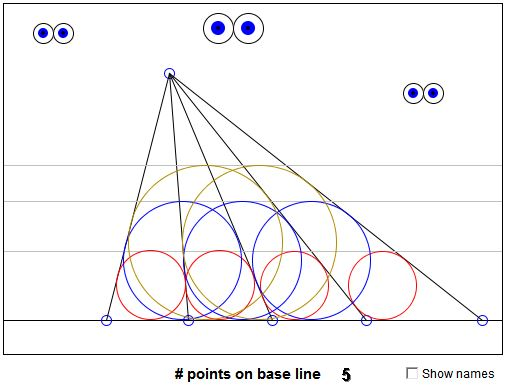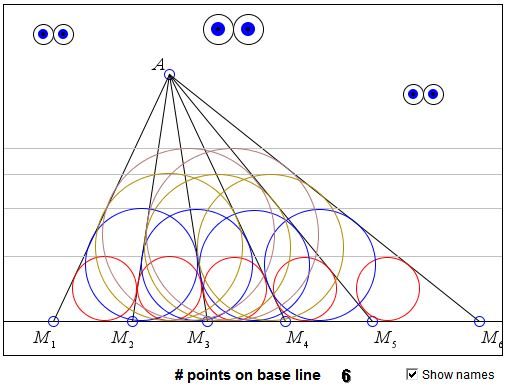Equal Incircles TheoremWhat Is It About? A Mathematical Droodle

You probably do not see the applet because nowadays no browser supports Java. Hopefully a solution could eventually be found. Meanwhile, here's what you would see if the applet was working:What if applet does not run?

(The number of points on the base line which is originally 4 can be changed by clicking on it a little off its center line.)

ExplanationEqual Incircles Theorem

The applet suggests the following theorem [Wells, p. 67] from triangle geometry:

Let A be a point. Assume points Mi, i = 1, 2, ..., N (N > 3) lie on a line not through A. Assume further that the incircles of triangles M1AM2, M2AM3, ..., MN-1AMN all have equal radii. Then the same is true of triangles M1AM3, M2AM4, ..., MN-2AMN, and also of triangles M1AM4, M2AM5, ..., MN-3AMN, and so on.I do not know of an elegant proof of that theorem. In [Wells, p. 67] it appears without a proof. There's no reference either.

The problem has been also discussed by R. Honsberger [Delights, section 17], where he mistakenly claims that the equality of the incircles only extends on the "triangles formed by sets of 2n consecutive triangles in the fan". The solution, nonetheless, works for a more general statement. Honsberger found this to be #2.2.5 in the Sangaku collection by H. Fukagawa and D. Pedoe. In fact problem #2.2.5 shows only two circles and the question is to find the length of the common tangent from the apex A in case the two circles are equal. In this form the problem appears on a surviving 1897 tablet from the Chiba prefecture. The answer to this problem can be found to be

AM2 = s(s - a),

where a is the base and s is the semiperimeter of the triangle.

However, the theorem is a consequence (and also a generalization) of a theorem published in 1986 by H. Demir, which, as was shown by J. B. Tabov, admits if not a more elegant proof, then quite an elegant generalization:

Assume n > 4, and the inradii of the "first level" triangles are equal in pairs: the inradius of ΔMiAMi+1 is equal to that of ΔMi+2AMi+3, i = 1, 2, ..., n-3. Then the incircles of the "second level" triangles MiAMi+2 are all equal.

(There is another, simple proof of the general statement.)

References

1. H. Fukagawa, D. Pedoe, Japanese Temple Geometry Problems, The Charles Babbage Research Center, Winnipeg, 1989
2. R. Honsberger, Mathematical Delights, MAA, 2004
3. D. Wells, Curious and Interesting Geometry, Penguin Books, 1991Sangaku